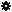﻿ Object Model: Vertex.NeighborPolygons

Vertex.NeighborPolygons

## Vertex.NeighborPolygonsv3.0

### Description

Returns a collection of PolygonFace objects for all polygon neighbors within the specified distance. If the distance is 1 all PolygonFace for which one of the Vertex objects is the current Vertex are returned in counter-clockwise order.

### C# Syntax

 ```PolygonFaceCollection Vertex.NeighborPolygons( Int32 in_lDistance ); ```

### Scripting Syntax

 ```oReturn = Vertex.NeighborPolygons( [Distance] ); ```

### Parameters

Parameter Type Description
Distance Integer Distance is an integer value representing the degree of neighborhood (eg: degree=2 for a vertex means its adjacent polygons plus the adjacent polygons of the adjacent polygons).

Default Value: 1

### Examples

#### VBScript Example

 ```set oCube = ActiveSceneRoot.AddGeometry( "Cube", "MeshSurface" ) set oGeometry = oCube.ActivePrimitive.Geometry for each Vertex in oGeometry.Vertices set oNeighbors = Vertex.NeighborPolygons str = "Polygons neighbors of Vertex(" & Vertex.index & ") are :" for each n in oNeighbors str = str & " " & n.index next logmessage str next ```